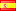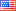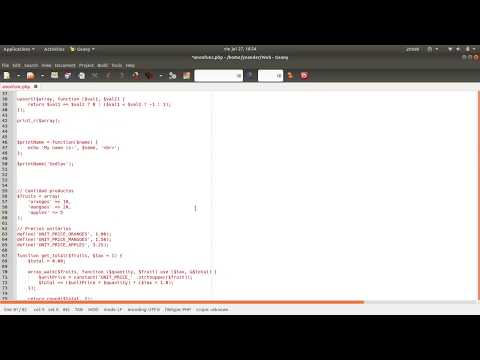# Anonymous functions in PHPWith the release of version 5.3.0, PHP development team incorporated what are called anonymous functions or closure.

An anonymous function, as its name implies, is nothing more than a function that has no name and that can be used as a callback allowing greater elegance and readability of source code, it is also possible to assign an anonymous function to a variable as were another data PHP type. PHP internally converts this variable in an instance of the Closure class.

##### Examples

1. Let's have the following array and want to sort them using uasort

```\$array = array(
'a' => 4,
'b' => 8,
'c' => -1,
'd' => -9,
'e' => 2,
'f' => 5,
'g' => 3,
'h' => -4
);
```

Before php-5.3.0 would have something like

```function compare(\$val1, \$val2)
{
return \$val1 == \$val2 ? 0 : (\$val1 < \$val2 ? -1 : 1);
}

\$array = array(
'a' => 4,
'b' => 8,
'c' => -1,
'd' => -9,
'e' => 2,
'f' => 5,
'g' => 3,
'h' => -4
);

uasort(\$array, 'compare');

print_r(\$array);

// Result
//Array
//(
//  [d] = -9
//  [h] = -4
//  [c] = -1
//  [e] = 2
//  [g] = 3
//  [a] = 4
//  [f] = 5
//  [b] = 8
//)
```

From php-5.3.0 using anonymous functions

```\$array = array(
'a' => 4,
'b' => 8,
'c' => -1,
'd' => -9,
'e' => 2,
'f' => 5,
'g' => 3,
'h' => -4
);

uasort(\$array, function (\$val1, \$val2) {
return \$val1 == \$val2 ? 0 : (\$val1 < \$val2 ? -1 : 1);
});

print_r(\$array);

// Result
//Array
//(
//  [d] = -9
//  [h] = -4
//  [c] = -1
//  [e] = 2
//  [g] = 3
//  [a] = 4
//  [f] = 5
//  [b] = 8
//)

```

Both examples produce the same result, but the second case allows a better organization and readability in source code.
2. Assign an anonymous function to a variable

```\$print_name = function (\$name)
{
echo 'My name is ', \$name, PHP_EOL;
};

\$print_name('Sedlav');
\$print_name('Jhon Doe');

// Print
// My name is Sedlav
// My name is Jhon Doe
```

Note the semicolon after the brace that closes the anonymous function, which is needed due to is one assignment more; If it is not a syntax error is generated.

3. Scope of anonymous functions

Anonymous functions can inherit variables from the parent context, the parent context is where the anonymous function was declared and not necessarily from where it is called; within an anonymous function you also can refer to constants and global variables.

```// Quantity products
\$products = array(
'oranges' => 10,
'mangoes' => 20,
'apples' => 5
);

// Unit prices
define('UNIT_PRICE_ORANGES', 1.00);
define('UNIT_PRICE_MANGOES', 1.50);
define('UNIT_PRICE_APPLES', 3.25);

function get_total(\$products, \$tax = 1) {
\$total = 0.00;

array_walk(\$products, function (\$quantity, \$product) use (\$tax, &\$total) {
\$pricePerItem = constant('UNIT_PRICE_' .strtoupper(\$product));
\$total += (\$pricePerItem * \$quantity) * (\$tax + 1.0);
});

return round(\$total, 2);
}

// Print the total price with a 5% tax
echo get_total(\$products, 0.05), PHP_EOL;

// The result is 59.06
//
```### PHP new features, 1 (8)

This site uses Akismet to reduce spam. Learn how your comment data is processed.